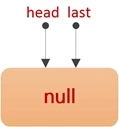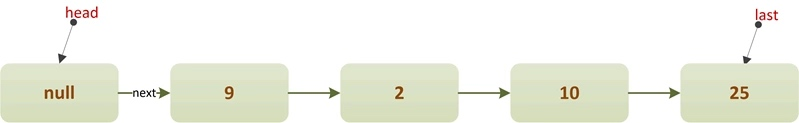Posted by 陈树义 on 2021-06-20``````LinkedBlockingQueue linkedBlockingQueue = new LinkedBlockingQueue();
``````

## 特性

• 1、底层使用链表实现。
• 2、只支持非公平策略，不支持公平策略。
• 3、使用两把锁takeLock和putLock控制并发，takeLock用于控制出队的并发，putLock用于入队的并发。可以同时有一个线程进行入队操作，一个线程进行出队操作。

## 原理

### 类成员变量

``````/** The capacity bound, or Integer.MAX_VALUE if none */
private final int capacity;

/** Current number of elements */
private final AtomicInteger count = new AtomicInteger();

/**
*/

/**
* Invariant: last.next == null
*/
private transient Node<E> last;

/** Lock held by take, poll, etc */
private final ReentrantLock takeLock = new ReentrantLock();

/** Wait queue for waiting takes */
private final Condition notEmpty = takeLock.newCondition();

/** Lock held by put, offer, etc */
private final ReentrantLock putLock = new ReentrantLock();

/** Wait queue for waiting puts */
private final Condition notFull = putLock.newCondition();
``````

``````static class Node<E> {
E item;

/**
* One of:
* - the real successor Node
* - this Node, meaning the successor is head.next
* - null, meaning there is no successor (this is the last node)
*/
Node<E> next;

Node(E x) { item = x; }
}
``````

### 构造方法

``````/**
* 默认构造器.
* 队列容量为Integer.MAX_VALUE.
*/
this(Integer.MAX_VALUE);
}
``````
``````/**
* 显示指定队列容量的构造器
*/
if (capacity <= 0) throw new IllegalArgumentException();
this.capacity = capacity;
last = head = new Node<E>(null);
}
``````
``````/**
* 从已有集合构造队列.
* 队列容量为Integer.MAX_VALUE
*/
public LinkedBlockingQueue(Collection<? extends E> c) {
this(Integer.MAX_VALUE);
final ReentrantLock putLock = this.putLock;
putLock.lock();                     // 这里加锁仅仅是为了保证可见性
try {
int n = 0;
for (E e : c) {
if (e == null)              // 队列不能包含null元素
throw new NullPointerException();
if (n == capacity)          // 队列已满
throw new IllegalStateException("Queue full");
enqueue(new Node<E>(e));    // 队尾插入元素
++n;
}
count.set(n);                   // 设置元素个数
} finally {
putLock.unlock();
}
}
``````### 核心方法

• offer/poll
• put/take

``````public boolean add(E e) {
}
``````

``````public boolean add(E e) {
if (offer(e))
return true;
else
throw new IllegalStateException("Queue full");
}
``````

``````public boolean remove(Object o) {
if (o == null) return false;
fullyLock();
try {
for (Node<E> trail = head, p = trail.next;
p != null;
trail = p, p = p.next) {
if (o.equals(p.item)) {
return true;
}
}
return false;
} finally {
fullyUnlock();
}
}
``````

#### offer/poll方法

``````public boolean offer(E e) {
if (e == null) throw new NullPointerException();
final AtomicInteger count = this.count;
if (count.get() == capacity)
return false;
int c = -1;
Node<E> node = new Node<E>(e);
final ReentrantLock putLock = this.putLock; // 1.获取写锁
putLock.lock();
try {
if (count.get() < capacity) {
enqueue(node);
c = count.getAndIncrement(); // c 表示入队前的队列元素个数
if (c + 1 < capacity)   // 2.唤醒等待插入的线程
notFull.signal();
}
} finally {
putLock.unlock();
}
if (c == 0) // 3.唤醒等待获取元素的线程
signalNotEmpty();
return c >= 0;
}
``````

``````private void enqueue(Node<E> node) {
// assert last.next == null;
last = last.next = node;
}
``````

``````if (c + 1 < capacity)   // CASE 1：唤醒等待插入的线程
notFull.signal();
``````

``````if (c == 0) // CASE 2：唤醒等待获取元素的线程
signalNotEmpty();
``````

``````private void signalNotEmpty() {
final ReentrantLock takeLock = this.takeLock;
takeLock.lock();
try {
notEmpty.signal();
} finally {
takeLock.unlock();
}
}
``````

``````public E poll() {
final AtomicInteger count = this.count;
if (count.get() == 0)
return null;
E x = null;
int c = -1;
final ReentrantLock takeLock = this.takeLock;
takeLock.lock();    // 1.获取出队锁
try {
if (count.get() > 0) {
x = dequeue();
c = count.getAndDecrement();
if (c > 1)  // 2.唤醒等待获取元素的线程
notEmpty.signal();
}
} finally {
takeLock.unlock();
}
if (c == capacity)  // 3.唤醒等待插入的线程
signalNotFull();
return x;
}
``````

``````private E dequeue() {
Node<E> first = h.next;
h.next = h; // help GC
E x = first.item;
first.item = null;
return x;
}
``````

``````if (c > 1)  // 2.唤醒等待获取元素的线程
notEmpty.signal();
``````

``````if (c == capacity)  // CASE 4：唤醒等待插入的线程
signalNotFull();
``````

signalNotFull 方法的内容与 signalNotEmpty 类似，都是先获取锁再唤醒。

``````private void signalNotFull() {
final ReentrantLock putLock = this.putLock;
putLock.lock();
try {
notFull.signal();
} finally {
putLock.unlock();
}
}
``````

#### put/take方法

``````public void put(E e) throws InterruptedException {
if (e == null) throw new NullPointerException();
// Note: convention in all put/take/etc is to preset local var
// holding count negative to indicate failure unless set.
int c = -1;
Node<E> node = new Node<E>(e);
final ReentrantLock putLock = this.putLock;
final AtomicInteger count = this.count;
putLock.lockInterruptibly();
try {
while (count.get() == capacity) {
notFull.await();
}
enqueue(node);
c = count.getAndIncrement();
if (c + 1 < capacity)   // CASE 1：唤醒其他正在等待插入的线程
notFull.signal();
} finally {
putLock.unlock();
}
if (c == 0)     // CASE 2：唤醒其他正在等待获取元素的线程
signalNotEmpty();
}
``````

put 方法的大致逻辑为：首先，获取入队锁（putLock）。接着，如果队列元素数量是否等于capacity，即队列是否已满。如果队列已满，那么在 notFull 队列等待，否则调用 enqueue 方法执行入队操作。这里调用的 enqueue 方法和 offer 方法中调用的 enqueue 方法是一样的，都是进行简单的引用修改。

``````private void enqueue(Node<E> node) {
// assert last.next == null;
last = last.next = node;
}
``````

``````if (c + 1 < capacity)   // CASE 1：唤醒其他正在等待插入的线程
notFull.signal();
``````

``````if (c == 0)     // CASE 2：唤醒其他正在等待获取元素的线程
signalNotEmpty();
``````

``````public E take() throws InterruptedException {
E x;
int c = -1;
final AtomicInteger count = this.count;
final ReentrantLock takeLock = this.takeLock;
takeLock.lockInterruptibly();   // 1.获取锁
try {
while (count.get() == 0) {
notEmpty.await();
}
x = dequeue();
c = count.getAndDecrement();
if (c > 1)  // 2.唤醒等待获取元素的线程
notEmpty.signal();
} finally {
takeLock.unlock();
}
if (c == capacity)  // 3.唤醒等待插入元素的线程
signalNotFull();
return x;
}
``````

take 方法的大致逻辑为：首先，获取出队锁（takeLock）。接着，判断队列大小是否等于0，即队列是否为空。如果队列为空，那么阻塞等待。否则调用 dequeue 方法进行出列操作。这里的 dequeue 方法与 poll 方法调用的 dequeue 方法是同一个方法。

``````private E dequeue() {
Node<E> first = h.next;
h.next = h; // help GC
E x = first.item;
first.item = null;
return x;
}
``````

``````if (c > 1)  // 2.唤醒等待获取元素的线程
notEmpty.signal();
``````

``````if (c == capacity)  // 3.唤醒等待插入元素的线程
signalNotFull();
``````

## 总结